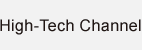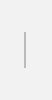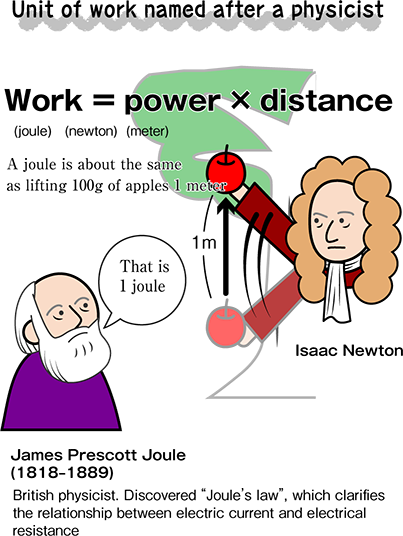### How much energy do you put into your work?

Symbol: J / Base data: energy, work, calorie. SI-derived unit.
Work done on an object when a force of 1N moves that object in the direction of the force's motion for a distance of 1 meter.

Expressed in “newton” and “distance”

Energy of course is needed to lift an object 1m. More energy is required to lift that object 2m. The unit that indicates this energy is called a “joule”. Work on an object when a force of 1 N moves that object for a distance of 1 m is equal to 1 joule.